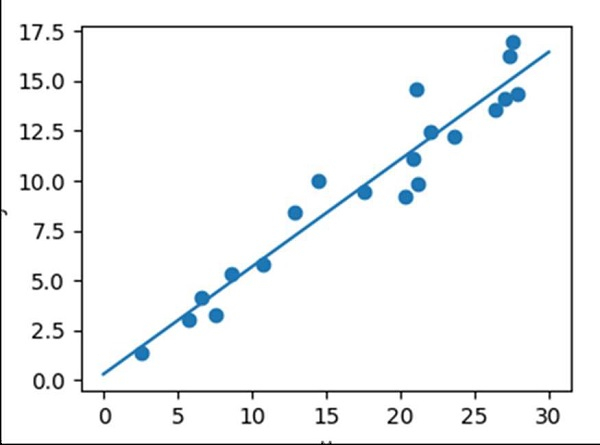# Linear regression with Matplotlib/Numpy

NumpyMatplotlibServer Side ProgrammingProgramming

To get a linear regression plot, we can use sklearn’s Linear Regression class, and further, we can draw the scatter points.

## Steps

• Get x data using np.random.random((20, 1)). Return random floats in the half-open interval[20, 1).

• Get the y data using np.random.normal() method. Draw random samples from a normal (Gaussian) distribution.

• Get ordinary least squares Linear Regression, i.e., model.

• Fit the linear model.

• Return evenly spaced numbers over a specified interval, using linspace() method.

• Predict using the linear model, using predict() method.

• Create a new figure, or activate an existing figure, with a given figsize tuple (4, 3).

• Add an axis to the current figure and make it the current axes, using axes() method.

• A scatter plot of *y* vs. *x* with varying marker size and/or color.

• Plot the line using x_new and y_new obtained from step 5 and 6.

• Set the X-axis label using plt.xlabel() method.

• Set the Y-axis label using plt.ylabel() method.

• Adjust the axis properties using ax.axis('tight').

• To show the figure, use plt.show() method.

## Example

import numpy as np
import matplotlib.pyplot as plt
from sklearn.linear_model import LinearRegression

x = 30 * np.random.random((20, 1))

y = 0.5 * x + 1.0 + np.random.normal(size=x.shape)

model = LinearRegression()
model.fit(x, y)

x_new = np.linspace(0, 30, 100)
y_new = model.predict(x_new[:, np.newaxis])

plt.figure(figsize=(4, 3))
ax = plt.axes()
ax.scatter(x, y)
ax.plot(x_new, y_new)

ax.set_xlabel('x')
ax.set_ylabel('y')

ax.axis('tight')

plt.show()

## Output